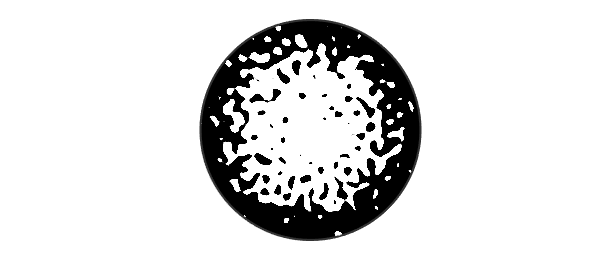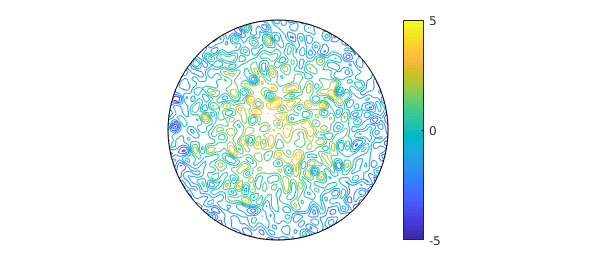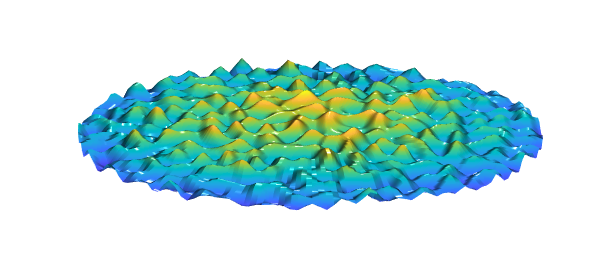Here is a smooth random function on the unit disk,

rng(1), random = randnfundisk(0.1);

and here is a paraboloid on the same domain,

paraboloid = diskfun(@(theta,r) 2-4*r.^2,'polar');

If we plot the sum of the two in zebra mode, we get an interesting picture:

f = random + paraboloid;
plot(f,'zebra'), axis equal offOf course zebra mode isn't the only way to plot a function. Here is a contour plot:

contour(f), colorbar, colormap('default'), axis offAnd here is a surface plot:

surf(f), zlim([-10 10])
camlight, camlight
view(0,60), axis offThe smooth random functions produced by randnfundisk are defined by finite Fourier series with random coefficients; see . As discussed in Section 7 of that paper, random surfaces have been studied since Longuet-Higgins in 1957 , and application areas include oceanography , biology , cosmology [2,6,9], condensed matter physics , and the melting of the Arctic . There is also interest among pure mathematicians  and other theoretical physicists . Chebfun's smooth random functions are examples of Gaussian random fields .

Our choice in this example to show random functions on a disk is arbitrary. Good times can also be had with randnfun, randnfun2, and randnfunsphere.

 R. J. Adler and J. E. Taylor, Random Fields and Geometry, Springer, 2009.

 J. M. Bardeen, J. R. Bond, N. Kaiser, and A. S. Szalay, The statistics of peaks of Gaussian random fields, Astrophys. J. 304 (1986), 15--61.

 E. Bogomolny and C. Schmit, Random wavefunctions and percolation, J. Phys. A 40 (2007), 14033--14043.

 B. Bowen, C. Strong, and K. M. Golden, Modeling the fractal geometry of Arctic melt pounds using the level sets of random surface, J. Fractal Geom. 5 (2018), 121--142.

 A. J. Bray and D. S. Dean, Statistics of critical points of Gaussian fields on large-dimensional spaces, Phys. Rev. Lett. 98 (2007), art. 150201.

 R. Easther, A. H. Guth, and A. Masoumi, Counting vacua in random landscapes, arXiv:1612.05224 (2016).

 S. Filip, A. Javeed, and L. N. Trefethen, Smooth random functions, random ODEs, and Gaussian processes, SIAM Rev. 61 (2019), 185--205.

 M. S. Longuet-Higgins, The statistical analysis of a random, moving surface, Phil. Trans. Roy. Soc. Lond. A 429 (1957), 321--387.

 J. Peacock, Cosmological Physics, Cambridge, 1999.

 A. Swishchuk and J. Wu, Evolution of Biological Systems in Random Media, Springer, 2013.